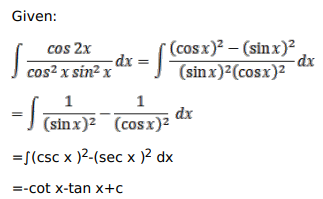# Solve this following

Question:

Mark $(\sqrt{)}$ against the correct answer in each of the following:

$\int \frac{\cos 2 x}{\cos ^{2} x \sin ^{2} x} d x=?$

A. $-\cot x-\tan x+C$

B. $-\cot x+\tan x+C$

C. $\cot x-\tan x+C$

D. $\cot x+\tan x+C$

Solution: